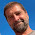Tuesday, November 24, 2015

Random Numbers in Java: Comparison of java.util.random, Xorshift, and PCG

In a previous post, we have shown how to replace the Java random number generator with a faster Xorshift algorithm, which also has nicer properties than the Java random number generator which was implemented in 1995.

Melissa E. O’Neill from Harvey Mudd College suggests a new class of random number generators called PCG. For a detailed description, see her paper "PCG: A Family of Simple Fast Space-Efficient Statistically Good Algorithms for Random Number Generation".

Like the Xorshift algorithm, PCG is using a couple of shift and bitwise logical operators to generate random integers. Typically this is faster that Java's linear congruential generator. We have implemented a simple version of the PCG algorithm in order to compare its performance to java.util.random and our Xorshift implementation.

The best way to replace the Java random generator in our opinion is to make a subclass of java.util.random and to overwrite the `next()` method. For Xorshift, the algorithm is as follows:

``````import java.util.Random;

/**
* A subclass of java.util.random that implements the
* Xorshift random number generator
*/

public class XSRandom extends Random {
private long seed;

public XSRandom(long seed) {
this.seed = seed;
}

protected int next(int nbits) {
long x = seed;
x ^= (x << 21);
x ^= (x >>> 35);
x ^= (x << 4);
seed = x;
x &= ((1L << nbits) - 1);
return (int) x;
}
}
``````

We implemented the PCG algorithm in Java based on the minimal C code example by M.E. O'Neill at pcg-random.org:

``````import java.util.Random;

/**
* A subclass of java.util.random that implements the
* PCG32 random number generator
* Based on the minimal code example by M.E. O'Neill / pcg-random.org
*/

public class PCGRandom extends Random {
private long inc;
private long state;

public PCGRandom(long seed) {
this.state = seed;
inc=1;
}

public PCGRandom(long seed, long initseq) {
// initseq selects the output sequence for the RNG
this.state = seed;
this.inc=initseq;
}

protected int next(int nbits) {
long oldstate=state;
state=oldstate * 6364136223846793005L + (inc | 1);
// Calculate output function (XSH RR), uses old state for max ILP
long xorshifted = ((oldstate >> 18) ^ oldstate) >> 27;
long rot = oldstate >> 59;
return (int) ((xorshifted >> rot) | (xorshifted << ((-rot) & 31)));
}
}
``````

To use the class in legacy code, you may also instantiate an `XSRandom` object and assign it to a java.util.Random variable:
``  java.util.Random rand = new XSRandom(); // or new PCGRandom``
All method calls to `rand `are then using the new implementation.

The PCG algorithm comes with better properties regarding its period. But what about the performance?
We tested the performance by calculating 107 random numbers subsequently, repeating this 10 times with different starting seeds. Then we compared the average execution time for a program compiled under Java 8 running single threaded on an core i7 notebook computer. Results show that for our implementation, the PCG algorithm has a very similar execution time to the original Java random generator, while the Xorshift is significantly faster.Execution time of java.util.random, Xorshift, and PCG. Please note that y axis starts at 10 ns.
So if you mainly care about speed, go for Xorshift. If statistical quality is important, go for PCG. If you want a bad random number generator and have a lot of time, stick with java.util.random.

1 comment:

1.If you want an excellent algorithm that's faster than PCG, better than pure xorshift, and has a period of 2^128, go width xorshift128+. It's what Google's V8 JavaScript engine is using now for Math.random(). You can find details at http://prng.di.unimi.it/